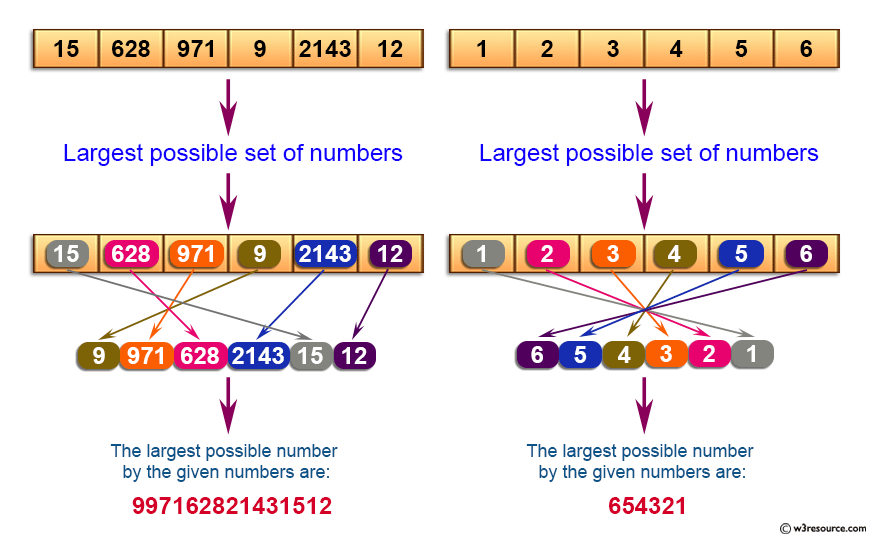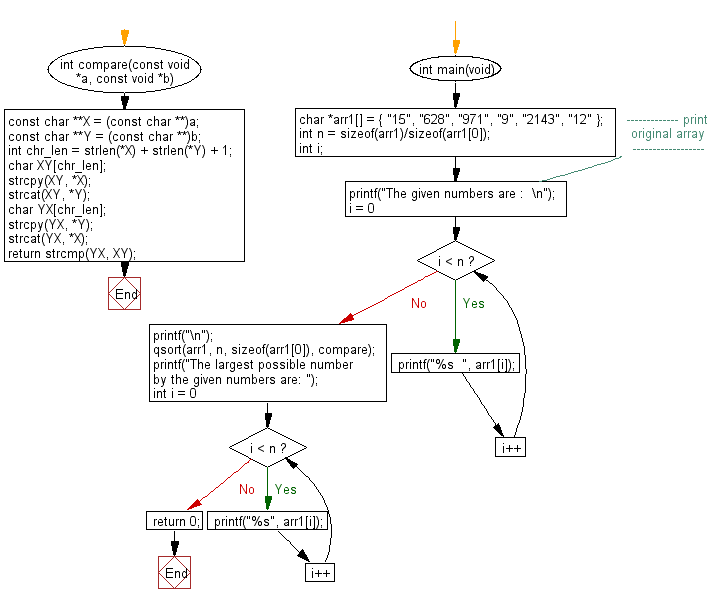﻿ C exercises: Find largest number possible from the set of specified numbers - w3resource# C Exercises: Find largest number possible from the set of specified numbers

## C Array: Exercise-76 with Solution

Write a program in C to find largest number possible from the set of given numbers.

Pictorial Presentation:Sample Solution:

C Code:

``````#include <stdio.h>
#include <string.h>
#include <stdlib.h>

int compare(const void *a, const void *b)
{
const char **X = (const char **)a;
const char **Y = (const char **)b;

int chr_len = strlen(*X) + strlen(*Y) + 1;

char XY[chr_len];
strcpy(XY, *X);
strcat(XY, *Y);

char YX[chr_len];
strcpy(YX, *Y);
strcat(YX, *X);

return strcmp(YX, XY);
}

int main(void)
{
char *arr1[] = { "15", "628", "971", "9", "2143", "12" };
int n = sizeof(arr1)/sizeof(arr1);
int i;
//------------- print original array ------------------
printf("The given numbers are :  \n");
for(i = 0; i < n; i++)
{
printf("%s  ", arr1[i]);
}
printf("\n");
//------------------------------------------------------
qsort(arr1, n, sizeof(arr1), compare);
printf("The largest possible number by the given numbers are: ");
for (int i = 0; i < n ; i++ )
printf("%s", arr1[i]);
return 0;
}
```
```

Sample Output:

```The given numbers are :
15  628  971  9  2143  12
The largest possible number by the given numbers are: 997162821431512
```

Flowchart:C Programming Code Editor:

Improve this sample solution and post your code through Disqus.

What is the difficulty level of this exercise?

﻿